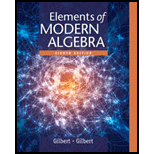# a. Let G = { [ a ] | [ a ] ≠ [ 0 ] } ⊆ ℤ n . Show that G is a group with respect to multiplication in ℤ n if and only if n is a prime. State the order of G . This group is called the group of units in ℤ n and is designated by U n . ( Sec 3.3 # 5 , Sec 3.4 # 11 , Sec 3.5 # 19 ) b . Construct a multiplication table for the group U 7 of all nonzero elements in ℤ 7 , and identify the inverse of each element. (Sec 4.4 , # 1 , 19 , 26 ) Sec 3.3 # 5 ≪ 5. Exercise 33 of section 3.1 shows that U 13 ⊆ ℤ 13 is a group under multiplication. List the elements of the subgroup 〈 [ 4 ] 〉 of U 13 , and state its order. List the elements of the subgroup 〈 [ 8 ] 〉 of U 13 , and state its order. Sec 3.4 # 11 ≪ 11. If n is a prime, the nonzero elements of ℤ n form a group U n with respect to multiplication. For each of the following values of n , show that this group U n is cyclic. n = 7 b . n = 5 c . n = 11 d . n = 13 e. n = 17 f. n = 19 Sec 3.5 # 19 ≪ 19. If n is a prime, U n , the set of nonzero elements of ℤ n , forms a group with respect to multiplication. Prove or disprove that the mapping ∅ : U n → U n defined by the rule in Exercise 18 is an automorphism of U n . Construct a multiplication table for the group U 7 of all nonzero elements in ℤ 7 , and identify the inverse of each element. (Sec 4.4 , # 1 , 19 , 26 ) Sec 4.4 , # 1 ≪ 1. Consider U 13 , the groups of units in ℤ 13 under multiplication. For each of the following subgroups H in U 13 , partition U 13 into left cosets of H , and state the index [ U 13 : H ] of H in U 13 H = 〈 [ 4 ] 〉 b. H = 〈 [ 8 ] 〉 Sec 4.4 , # 19 ≪ 19. Find the order of each of the following elements in the multiplicative group of units U p . [ 2 ] for p = 13 b. [ 5 ] for p = 13 c. [ 3 ] for p = 17 d . [ 8 ] for p = 17 Sec 4.4 , # 26 ≪ 26 . Let p be prime and G the multiplicative group of units U p = { [ a ] ∈ ℤ p | [ a ] ≠ [ 0 ] } . Use Langrange’s Theorem in G to prove Fermat’s Little Theorem in the form [ a ] p = [ a ] for any a ∈ ℤ .### Elements Of Modern Algebra

8th Edition
Gilbert + 2 others
Publisher: Cengage Learning,
ISBN: 9781285463230### Elements Of Modern Algebra

8th Edition
Gilbert + 2 others
Publisher: Cengage Learning,
ISBN: 9781285463230

#### Solutions

Chapter
Section
Chapter 3.1, Problem 33E
Textbook Problem

## Expert Solution

### Want to see the full answer?

Check out a sample textbook solution.See solution

### Want to see this answer and more?

Experts are waiting 24/7 to provide step-by-step solutions in as fast as 30 minutes!*

See Solution

*Response times vary by subject and question complexity. Median response time is 34 minutes and may be longer for new subjects.